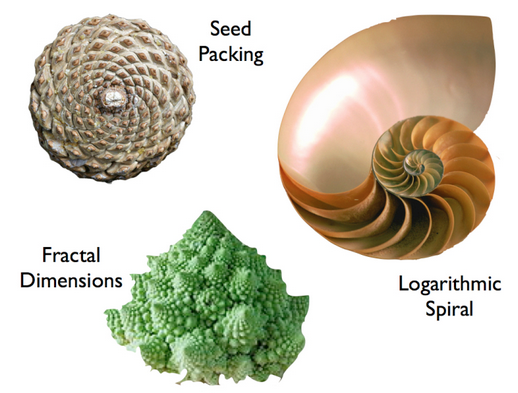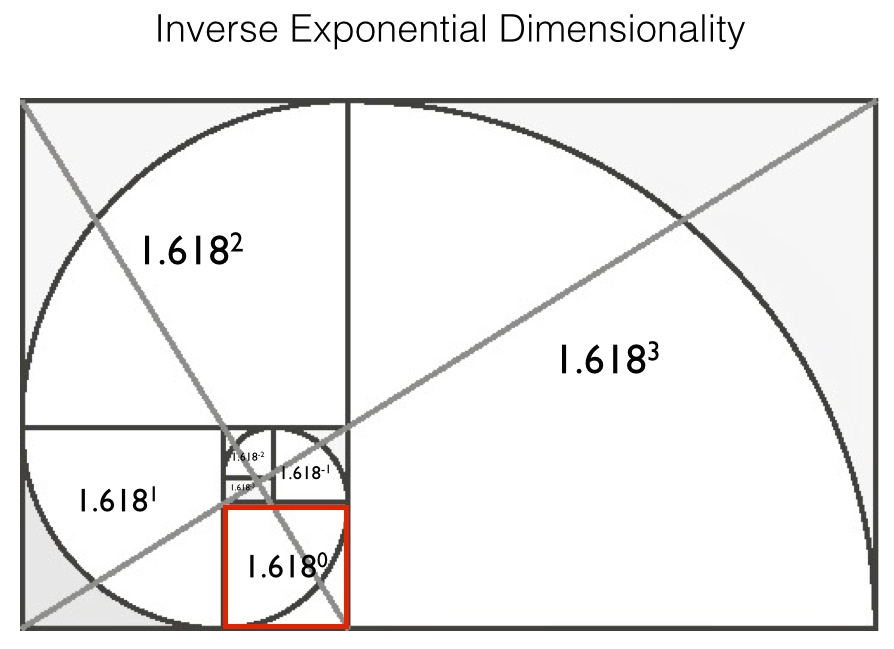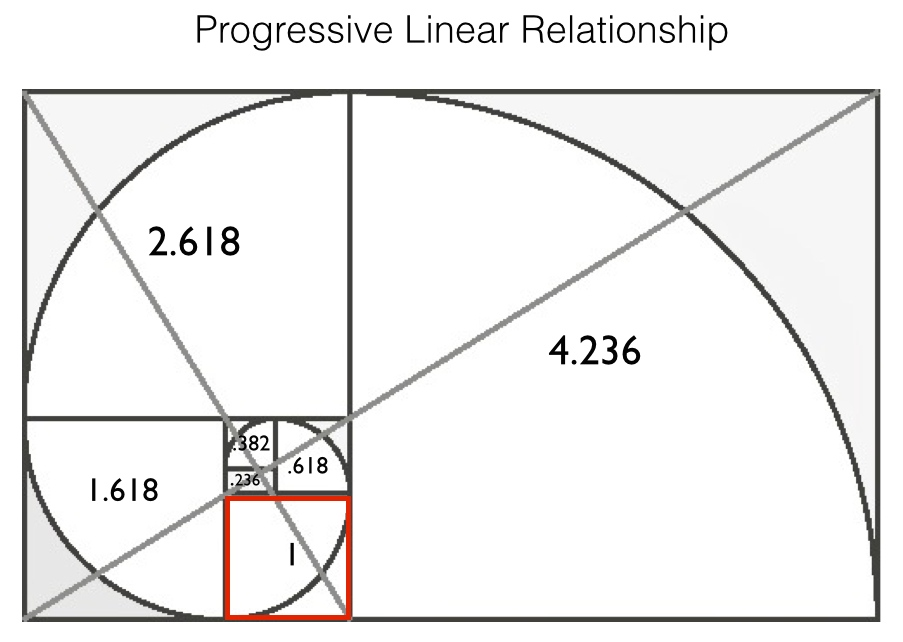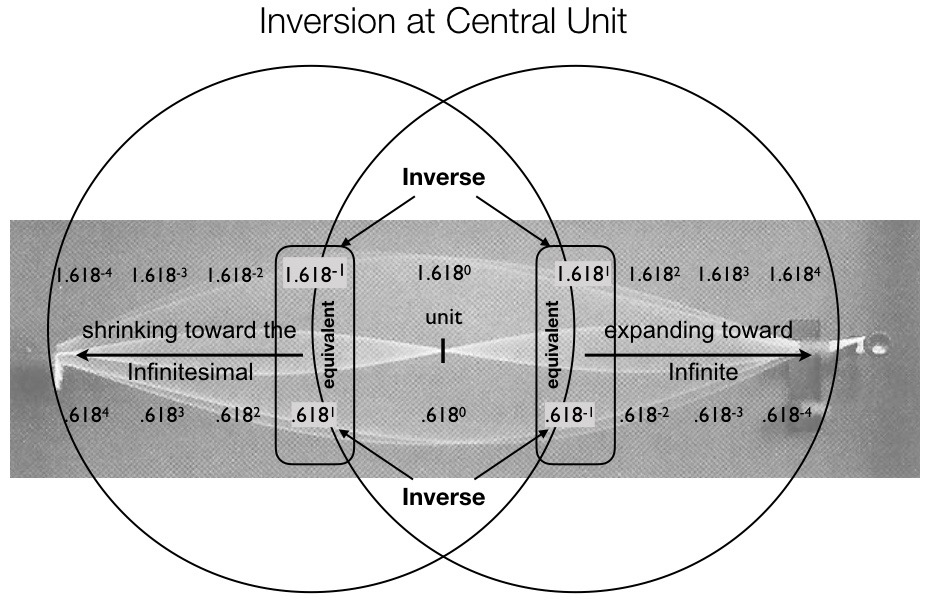# Aside: Geometry of Spin and Span

Seen below are circles of various sizes.  The larger the circle gets, the less curve (arc angle) its circumference has; in other words, the structure of the circle changes with exSpansion.Optimally, an E/S prefers to maintain its current Pattern/Structure while it reserves more Matter/Substance.

In the images below, observe the overlay of clockwise Spin (CWS) and counter clockwise Spin (CCWS).  See how the Spinning of radii condenses/consolidates Matter while it minimizes radial Span.  Notice how the overall Pattern/Structure is maintained despite growth in Matter/Substance.If boundary-closed (for maintenance) and boundary-open (for growth) were equal in value though opposite in sign or charge, they would negate each other and be ineffectual.  Think:  -1  +  1  = 0.

Open-boundary and closed-boundary states are inverse of each other; the more of one the less of the other.  The more a boundary is open the less it can be closed.

However, negative feedback and positive feedback are not like opposites that cancel each other.  Negative feedback does not destroy; it maintains.  Positive Feedback amplifies, providing for unhampered growth.

Rather than negating one another, maintenance and growth complement each other.  They do not relate as opposites that sum to zero, as in -1 +1 = 0, -64 + 64 = 0, etc.  Rather, they are in inverse proportion, so their product is always one, like this 1/2 x 2/1 = 1, 1/3 x 3/1 = 1, 1/2985 x 2985/1 = 1

When positive and negative feedback are in the perfect proportion there is a slow, steady and manageable growth/evolution.

#### Spin and Span are Inversely Proportional

If purely inverse, we would say the more Spin the less Span, and vice versa.  However, to accomplish both maintenance and growth, which are two opposite motivations, only one proportion will work.

As we learned from the Mathematical Models unit, a ‘golden’ proportion balances specific inverse values, like maintenance and growth.  This unique proportion involves a pair of irrational, transcendental numbers, called phi and Phi, or .618 and 1.618, respectively.

.618 is to 1 as 1 is to 1.618

To illustrate Spin and Span, we construct a rectangle with sides in the ‘golden’ proportion.  The sides can be either .618 and 1, or they can be 1 and 1.618; the proportion will be the same in either case.If you want to enlarge graphics for better viewing just click on them.

Within the ‘golden’ rectangle above is a logarithmic spiralThe Spin of the logarithmic spiral is such that the arc angle never changes, even as the squares Span, or grow/change/open-outwardly.

No other proportion in the universe provides this exquisite balance, and it is for this important reason — Phi/1.618 and phi/.618 are the only values that are both inverse of each other (check it out on your calculator!)…

1.618033989 x .618033989 = 1

AND that differ by the unitary value of one.  (You can do this in your head. 🙂 )

1.618033989 – .618033989 = 1.

Phi and phi performed as portals in the chapter Discovering Portals between our Dual Dimensions.In the rectangle reprinted above, orient yourself to the red square containing 1.618º.  Watch the exponents increase as the squares get larger, and decrease as the squares get smaller.  The exponents on either side of the center zero, are paired: -1 inside and +1 outside, -2 inside and + 2 outside, etc.  The smallest value has the highest negative exponent and the largest value has the highest positive exponent.  From least to greatest, the squares progress seamlessly (consistently by the same proportion), passing right through the square outlined in red without so much as a flutter.

In the rectangle below, each square contains the numerical value of the mathematical expression contained in the matching square of the rectangle above.  In other words, the expression 1.618º in the red square of the previous rectangle has a numerical value of 1, which is now shown in the matching red square of the rectangle below.  Any base number with a zero exponent acquires the value of one.

Orient yourself again to the red square in the rectangle below.  From the central value of 1, add the values in the boxes as they increase in size (1 + 1.618 = 2.618, etc.) or subtract the values as the boxes get smaller (1 – .618 = .382, etc.).  The numerical values, from smallest to largest, again flow seamlessly through the central unit.Quadernity’s Nature-Based Number Line taught us that there are no zeros in nature.  Every single thing can be counted as a (+1).  Whatever you designate as your “something” becomes the central-one.  Your Particularity (particular entity) will have Parts on the inside that shrink toward the infinitesimal, and it will Participate in an outside hierarchy that extends to infinity.  The graphic below, reprinted from the Number Line chapter, reveals Spin as “shrinking toward the infinitesimal” value and Span as “expanding toward the infinite” value.The Particularity, situated between the shrinking inside Parts (Spin) and expanding outside Participation (Span), is like a boundary whereupon Spin and Span are coterminous.  This does not mean that NEITHER Spin nor Span exist; instead, BOTH Spin and Span are temporarily matched, or aligned, to make a hand-off.   The OUTput of Span becomes the INput of Spin.

The negative and positive exponents related to the inequalities of Spin and Span may offset to a nil affect, but the Particularity always retains its +1 status.  The hierarchically organized entity/system (E/S) extends throughout its myriad dimensions by Spinning and Spanning.

As Phi and phi go to the power of 0 (in the center of graphic above), they become equal.  Indifferentiable, they are identified, each to the other; they are ONE from which countless pairs of inverse values emerge.

The rows of numbers in the graphic reprinted above are, column-by-column, equivalent in value; the upper value equals lower value, even though stated differently.  From left to right, the actual numerical values for each of the nine columns are as follows:

.146  .236  .382  .618  1  1.618  2.618  4.236  6.854

Take the first two values and add them to arrive at the third value.  Then add the second and third terms to get the fourth term.  Continue in this manner to form an Additive Summation Series:

• .146 + .236 = .382
• .236 + .382 = .618
• .382 + .618 = 1
• .618 + 1 = 1.618
• 1 + 1.618 = 2.618
• 1.618 + 2.618 = 4.236
• 2.618 + 4.236 = 6.854

The seamless flow, as demonstrated by the two golden rectangles above, happens on two levels: first, by Additive Summation Series (seen above) and second, by powers of Phi (seen below):

• .146 x 1.618 = .236
• .236 x 1.618 = .382
• .382 x 1.618 = .618
• .618 x 1.618 = 1
• 1 x 1.618 = 1.618
• 1.618 x 1.618 = 2.618
• 2.618 x 1.618 = 4.236
• 4.236 x 1.618 = 6.854

When Spin and Span are enjoined by a multi-dimensional E/S to perpetuate its existence, the linear, moment-to-moment integration of discrete data (as reflected in the Additive Summation Series) is perfectly aligned with the multi-dimensional Particularity (as reflected in the progressive powers of Phi).

Our own time-lines are linear, or one-dimensional, and yet we, too, are multi-dimensional beings.  When we walk across a room, we take linear steps in linear time; however, our hierarchically organized layers of Parts come along as we stride.  Moreover, our Participation in familial, social, political or religious organizations continue unabated as we move about.

Our 1D temporal experiences are so thoroughly identified with the interactivity among our multi-dimensional existence that many readers may be taken by surprise at this analysis.  Yet, it is through this kind of analysis that we can fully embrace the precision and profundity of nature-based mathematics.

Persistent patterns, sustainable systems, boundaries and orbits, all incorporate the logarithmic proportions of Spin and Span.Revealed in the images above is the logarithmic spiral, ubiquitous on every scale in the universe.

The Golden Proportion allows each Particularity to maintain its core essence.  Inward Spin constrains boundary, holding close to the center for maintenance, while it simultaneously draws resources inward, for growth.  While inward Spin maintains the arc angle, outward Span increases the filaments, seeds, leaves, or segments that fill the space.